NEET  >  31 Year NEET Previous Year Questions: Electromagnetic Waves

# 31 Year NEET Previous Year Questions: Electromagnetic Waves

Test Description

## 27 Questions MCQ Test Physics Class 12 | 31 Year NEET Previous Year Questions: Electromagnetic Waves

31 Year NEET Previous Year Questions: Electromagnetic Waves for NEET 2023 is part of Physics Class 12 preparation. The 31 Year NEET Previous Year Questions: Electromagnetic Waves questions and answers have been prepared according to the NEET exam syllabus.The 31 Year NEET Previous Year Questions: Electromagnetic Waves MCQs are made for NEET 2023 Exam. Find important definitions, questions, notes, meanings, examples, exercises, MCQs and online tests for 31 Year NEET Previous Year Questions: Electromagnetic Waves below.
Solutions of 31 Year NEET Previous Year Questions: Electromagnetic Waves questions in English are available as part of our Physics Class 12 for NEET & 31 Year NEET Previous Year Questions: Electromagnetic Waves solutions in Hindi for Physics Class 12 course. Download more important topics, notes, lectures and mock test series for NEET Exam by signing up for free. Attempt 31 Year NEET Previous Year Questions: Electromagnetic Waves | 27 questions in 54 minutes | Mock test for NEET preparation | Free important questions MCQ to study Physics Class 12 for NEET Exam | Download free PDF with solutions
 1 Crore+ students have signed up on EduRev. Have you?
31 Year NEET Previous Year Questions: Electromagnetic Waves - Question 1

### Which of the following, is the longest wave ?       

Detailed Solution for 31 Year NEET Previous Year Questions: Electromagnetic Waves - Question 1

Rays  -    Wavelength
[Range in m]
X-rays    -  1 × 10–11 to 3 × 10–8
γ-rays     -  6 × 10–14 to 1 × 10–11
Microwaves    -  10–3 to 0.3

31 Year NEET Previous Year Questions: Electromagnetic Waves - Question 2

### Pick out the longest wavelength from the following types of radiation. 

Detailed Solution for 31 Year NEET Previous Year Questions: Electromagnetic Waves - Question 2

Wavelength of red light is longest

31 Year NEET Previous Year Questions: Electromagnetic Waves - Question 3

### The frequency of electromagnetic wave, whichis best suited to observe a particle of radius3 × 10–4 cm is of the order of             

Detailed Solution for 31 Year NEET Previous Year Questions: Electromagnetic Waves - Question 3

The Wave length of radiation used should be less than the size of the particle =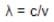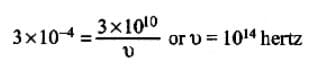31 Year NEET Previous Year Questions: Electromagnetic Waves - Question 4

The structure of solids is investigated by using        

Detailed Solution for 31 Year NEET Previous Year Questions: Electromagnetic Waves - Question 4

X-rays are used for the investigation of
structure of solids

31 Year NEET Previous Year Questions: Electromagnetic Waves - Question 5

A signal emitted by an antenna from a certainpoint can be received at another point of thesurface in the form of                

Detailed Solution for 31 Year NEET Previous Year Questions: Electromagnetic Waves - Question 5

Sea waves travel in a straight line thus, they can only be used for in line communication. Whereas sky waves and ground waves are used for larger distances.

31 Year NEET Previous Year Questions: Electromagnetic Waves - Question 6

The oscillating electric and magnetic field vectorsof electromagnetic wave are oriented along   

Detailed Solution for 31 Year NEET Previous Year Questions: Electromagnetic Waves - Question 6

The direction of oscillations of E and B fields are perpendicular to each other as well as to the direction of propagation. So, electromagnetic waves are transverse in nature. The electric and magnetic fields oscillate in same phase.

31 Year NEET Previous Year Questions: Electromagnetic Waves - Question 7

Which one of the following electromagneticradiations has the smallest wavelength? 

Detailed Solution for 31 Year NEET Previous Year Questions: Electromagnetic Waves - Question 7

Rays  -    Wavelength
[Range in m]
X-rays    -  1 × 10–11 to 3 × 10–8
γ-rays     -  6 × 10–14 to 1 × 10–11
Microwaves    -  10–3 to 0.3

31 Year NEET Previous Year Questions: Electromagnetic Waves - Question 8

If ∈0 and μ0 are the electric permittivity and  magnetic permeability in vacuum, ∈ and μ are corresponding quantities in medium, then refractive index of the medium is 

Detailed Solution for 31 Year NEET Previous Year Questions: Electromagnetic Waves - Question 8

We know that velocity of  lectromagnetic
wave in vacuum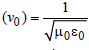velocity of electromagnetic wave in
medium is (v) =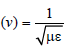Therefore refractive index of the medium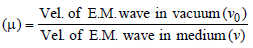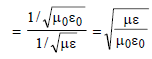31 Year NEET Previous Year Questions: Electromagnetic Waves - Question 9

​In a circuit, inductance L and capacitance C are connected as shown in the figure. A1 and A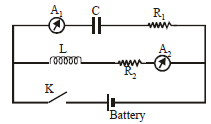are ammeters. When key K is pressed to complete the circuit, then just after closing key (K), the readings of A1 and A2 will be          

Detailed Solution for 31 Year NEET Previous Year Questions: Electromagnetic Waves - Question 9

Initially there is no D.C. current in inductive circuit and maximum D.C. current is in capacitive current. Hence, the current is zero in A2 and maximum in A1.

31 Year NEET Previous Year Questions: Electromagnetic Waves - Question 10

The electromagnetic radiations are caused by       

Detailed Solution for 31 Year NEET Previous Year Questions: Electromagnetic Waves - Question 10

A stationary charge produces electric field only; an uniformly moving charge produces localised electromagnetic field; an accelerated charge produces electromagnetic radiations.

31 Year NEET Previous Year Questions: Electromagnetic Waves - Question 11

The frequencies of X-rays, γ-rays and ultravioletrays are respectively a, b, and c. Then      

Detailed Solution for 31 Year NEET Previous Year Questions: Electromagnetic Waves - Question 11

γ rays has lowest wavelength and highest frequency among them while ultraviolet ray has highest wavelength and lowest frequency.
Order of frequency : b > a > c

31 Year NEET Previous Year Questions: Electromagnetic Waves - Question 12

Which of the following is positively charged?      

Detailed Solution for 31 Year NEET Previous Year Questions: Electromagnetic Waves - Question 12

α rays contain Helium nuclei which contains 2 unit of positive charge.

31 Year NEET Previous Year Questions: Electromagnetic Waves - Question 13

if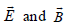represent electric and magnetic field vectors of the electromagnetic waves, then the direction of propagation of the waves will be along

Detailed Solution for 31 Year NEET Previous Year Questions: Electromagnetic Waves - Question 13

Direction of propagation of electro-magnetic waves is perpendicular to Electric field and Magnetic field. Hence, direction is given by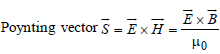31 Year NEET Previous Year Questions: Electromagnetic Waves - Question 14

Which of the following electromagneticradiations has the least wavelength? 

Detailed Solution for 31 Year NEET Previous Year Questions: Electromagnetic Waves - Question 14

Gamma ray has highest frequency and lowest wavelength.

31 Year NEET Previous Year Questions: Electromagnetic Waves - Question 15

Green-house effect is the heating up of earth's atmosphere due to         

Detailed Solution for 31 Year NEET Previous Year Questions: Electromagnetic Waves - Question 15

Infrared rays is the cause of Green house effect. The glass transmits visible light and short infrared rays which are absorbed by plants. Then it emits long infrared rays, which are reflected back by glass.

31 Year NEET Previous Year Questions: Electromagnetic Waves - Question 16

Which one of the following rays is notelectromagnetic wave ? 

Detailed Solution for 31 Year NEET Previous Year Questions: Electromagnetic Waves - Question 16

β ray is not electromagnetic ray

31 Year NEET Previous Year Questions: Electromagnetic Waves - Question 17

We consider the radiation emitted by the humanbody. Which of the following statements is true?      

Detailed Solution for 31 Year NEET Previous Year Questions: Electromagnetic Waves - Question 17

Depends on the magnitude of frequency

31 Year NEET Previous Year Questions: Electromagnetic Waves - Question 18

If λv, λx and λm represent the wavelengths of visible light, X–rays and microwaves respectively, then

Detailed Solution for 31 Year NEET Previous Year Questions: Electromagnetic Waves - Question 18

We know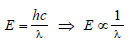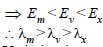31 Year NEET Previous Year Questions: Electromagnetic Waves - Question 19

The electric and magnetic field of an electromagneticwave are       

Detailed Solution for 31 Year NEET Previous Year Questions: Electromagnetic Waves - Question 19

Variation in magnetic field causes electric field and vice-versa.
In electromagnetic waves,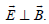.
Both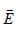and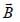are in the same phase.
In electromagnetic waves
E = E0 sin(ωt - kx)
B = B0 sin(ωt - kx)
The electromagnetic waves travel in the
direction of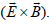31 Year NEET Previous Year Questions: Electromagnetic Waves - Question 20

The velocity of electromagnetic radiation in a medium of permittivity ∈0 and permeability μ0 is given by 

Detailed Solution for 31 Year NEET Previous Year Questions: Electromagnetic Waves - Question 20

in a medium of permittivity  ∈and
permeability μis equal to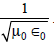31 Year NEET Previous Year Questions: Electromagnetic Waves - Question 21

The electric field part of an electromagnetic wave in a medium is represented by Ex=0;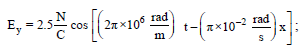Ez = 0. The wave is :               

Detailed Solution for 31 Year NEET Previous Year Questions: Electromagnetic Waves - Question 21

Comparing with the equation of wave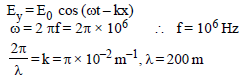31 Year NEET Previous Year Questions: Electromagnetic Waves - Question 22

Which of the following statement is false for theproperties of electromagnetic waves? 

Detailed Solution for 31 Year NEET Previous Year Questions: Electromagnetic Waves - Question 22

Electromagnetic waves are the combination of mutually perpendicular electric and magnetic fields. So, option (c) is false.

31 Year NEET Previous Year Questions: Electromagnetic Waves - Question 23

The electric and the magnetic field associated with an E.M. wave, propagating along the +z-axis, can be represented by 

Detailed Solution for 31 Year NEET Previous Year Questions: Electromagnetic Waves - Question 23

The electromagnetic wave is propagating along the +z axis.
Since the electric and magnetic fields are perpendicular to each other and also perpendicular to the direction of propagation of wave.
Also.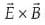gives the direction of wave propagation.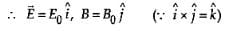31 Year NEET Previous Year Questions: Electromagnetic Waves - Question 24

The decreasing order of wavelength of infrared,microwave, ultraviolet and gamma rays is 

Detailed Solution for 31 Year NEET Previous Year Questions: Electromagnetic Waves - Question 24

The decreasing order of the wavelengths is as given below :
microwave, infrared, ultraviolet, gamma
rays.

31 Year NEET Previous Year Questions: Electromagnetic Waves - Question 25

The electric field associated with an e.m. wave in vacuum is given by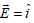cos (kz – 6 ×108t), where E, z and t are in volt/m, meter and seconds respectively. The value of wave vector k is : 

Detailed Solution for 31 Year NEET Previous Year Questions: Electromagnetic Waves - Question 25

On comparing the given equation to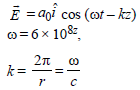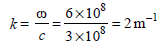31 Year NEET Previous Year Questions: Electromagnetic Waves - Question 26

The ratio of amplitude of magnetic field to theamplitude of electric field for an electromagneticwave propagating in vacuum is equal to :   [2012M]

Detailed Solution for 31 Year NEET Previous Year Questions: Electromagnetic Waves - Question 26

The average energy stored in the electric field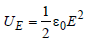The average energy stored in the magnetic field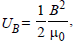According to conservation of energy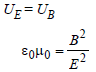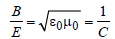31 Year NEET Previous Year Questions: Electromagnetic Waves - Question 27

An electromagnetic wave of frequency v = 3.0MHz passes from vacuum into a dielectricmedium with relative permittivity ε = 4.0. Then[NEET Kar. 2013]

Detailed Solution for 31 Year NEET Previous Year Questions: Electromagnetic Waves - Question 27

Given: frequency f = 2MHz, relative
permittivity ∈r= 4

From formula,

velocity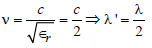[Since frequency remains unchanged]

## Physics Class 12

157 videos|452 docs|213 tests
 Use Code STAYHOME200 and get INR 200 additional OFF Use Coupon Code
Information about 31 Year NEET Previous Year Questions: Electromagnetic Waves Page
In this test you can find the Exam questions for 31 Year NEET Previous Year Questions: Electromagnetic Waves solved & explained in the simplest way possible. Besides giving Questions and answers for 31 Year NEET Previous Year Questions: Electromagnetic Waves, EduRev gives you an ample number of Online tests for practice

## Physics Class 12

157 videos|452 docs|213 tests

### How to Prepare for NEET

Read our guide to prepare for NEET which is created by Toppers & the best Teachers